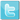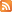Yara WorkBench

Author: David Zimmer
Date: 12.17.19 - 2:56am

Releasing a new tool today to help you develop and test Yara Signatures.

Yara Workbench is a full IDE with syntax highlighting, code completion, bench marking, match offsets, and in depth match details.

Since the initial video I had added in a couple more bonuses such as:
• module dump: (yara -D option)
• loads into a syntax highlight control
• if intVal > 9 dump values default to hex
• dll_imports[]{.name, .funcCount} fields
• launch selected file in an internal or external hex editor
• launch selected file in external disassembler

I also added two new functions to the pe module. pe.dbg(string) and pe.dbg(int). These can be used as a simple way to get some insight into how yara is running.

This can come in handy for example in the following scenario:
• pe.imphash() output is not included in the pe module dump
• a sample file has a corrupt import table
• what value does imphash() return? hint its not UNDEFINED or ""
With the new functions we can display its output with pe.dbg(pe.imphash()) to see the value of d41d8cd98f00b204e9800998ecf8427e which is md5("")

This could also be useful if a uint32() read was not returning what you expected, and wanted an easy way to see some debug output.

The dbg function will always return 1 so it can be used in the condition section without error.

Another useful example would be using it to get yara to dump pe section entropy:

import "pe"
import "math"

rule test
{
condition:
for all i in (0 .. pe.number_of_sections -1):(
pe.dbg(
pe.sections[i].name,
math.entropy(
pe.sections[i].raw_data_offset,
pe.sections[i].raw_data_size
)
)
)

}

Another example to see how yara handles corrupt import tables or resources:

rule dumpImportState
{
condition:
pe.dbg("imphash", pe.imphash()) and
pe.dbg("import_errors", pe.import_errors) and
pe.dbg("NumImports" , pe.number_of_imports-1) and
for all i in (0 .. pe.number_of_imports):(
pe.dbg( pe.dll_imports[i].funcCount, pe.dll_imports[i].name)
)
}

rule dumpResources
{
condition:
pe.dbg("NumResources" , pe.number_of_resources-1) and
for any i in (0 .. pe.number_of_resources-1):(
not pe.dbgw(pe.resources[i].name_string)
)
}

I hacked it into an existing module since the built in functions like uint32() get compiled into rules as binary opcodes and it just wasnt worth the fuss to add a native dbg() function at this point. Adding new functions to modules was quite easy below are the basic mods I used. (More details on the import_errors and dll_imports[] can be found in Yara git issue 1224)

//dzzie
declare_integer("import_errors");

begin_struct_array("dll_imports");
declare_string("name");
declare_integer("funcCount");
end_struct("dll_imports");

declare_function("dbgw", "s", "i", dbgw); //wide string version for resource names
declare_function("dbg", "s", "i", dbg);   //name,arg,retType,func
declare_function("dbg", "i", "i", dbgi);
declare_function("dbg", "f", "i", dbgf);
declare_function("dbg", "sf", "i", dbgsf);
declare_function("dbg", "si", "i", dbgsi);
declare_function("dbg", "is", "i", dbgis);
declare_function("dbg", "ii", "i", dbgii);
declare_function("dbg", "ss", "i", dbgss);

declare_function("isdef", "s", "i", isdef);
//dzzie

define_function(dbgw) { char buf = { 0 }; int i = 0, j = 0; char* name = string_argument(1); while (i < 253) { if (name[i] == 0 && name[i + 1] == 0) break; if (name[i] != 0) { buf[j++] = name[i]; } i++; } vb_dbg(cb_dbg, buf); return_integer(1); } define_function(dbg) { char* name = string_argument(1); vb_dbg(cb_dbg, name); return_integer(1); } define_function(dbgii) { char buf = { 0 }; int v = integer_argument(1); int z = integer_argument(2); snprintf(buf, sizeof(buf)-1, "0x%x 0x%x", v,z); vb_dbg(cb_dbg, buf); return_integer(1); } define_function(dbgss) { char buf = { 0 }; char* v = string_argument(1); char* z = string_argument(2); snprintf(buf, sizeof(buf)-1, "%s %s", v, z); vb_dbg(cb_dbg, buf); return_integer(1); } define_function(dbgi) { char buf = { 0 }; int v = integer_argument(1); if (v < 10) snprintf(buf, sizeof(buf)-1, "%d", v); else snprintf(buf, sizeof(buf)-1, "0x%x", v); vb_dbg(cb_dbg, buf); return_integer(1); } define_function(dbgf) { char buf = {0}; float v = float_argument(1); snprintf(buf, sizeof(buf)-1, "%.6f", v); vb_dbg(cb_dbg, buf); return_integer(1); } define_function(dbgsf) { char* txt = string_argument(1); float v = float_argument(2); int sz = strlen(txt) + 255; char* buf = (char*)malloc(sz); if (buf == NULL) { vb_dbg(cb_dbg, "dbgsf Failed to malloc %d bytes",sz); return_integer(1); } snprintf(buf, sz-1, "%s = %.6f", txt, v); vb_dbg(cb_dbg, buf); free(buf); return_integer(1); } define_function(dbgis) { char* txt = string_argument(2); int v = integer_argument(1); int sz = strlen(txt) + 255; char* buf = (char*)malloc(sz); if (buf == NULL) { vb_dbg(cb_dbg, "dbgsi Failed to malloc %d bytes", sz); return_integer(1); } snprintf(buf, sz-1, "0x%x = %s", v, txt); vb_dbg(cb_dbg, buf); free(buf); return_integer(1); } define_function(dbgsi) { char* txt = string_argument(1); int v = integer_argument(2); int sz = strlen(txt) + 255; char* buf = (char*)malloc(sz); if (buf == NULL) { vb_dbg(cb_dbg, "dbgsi Failed to malloc %d bytes", sz); return_integer(1); } snprintf(buf, sz-1, "%s = 0x%x", txt, v); vb_dbg(cb_dbg, buf); free(buf); return_integer(1); } //declare_function("isdef", "s", "i", isdef); //ex: pe.dbg(pe.isdef("rich_signature.offset")) // pe.dbg(pe.isdef("number_of_signatures")) define_function(isdef) { YR_OBJECT* module = module(); char* txt = string_argument(1); if (yr_object_has_undefined_value(module, txt)){ return_integer(0); }else{ return_integer(1); } }

Another one i found I wanted:

declare_function("section_exists", "s", "i", section_name_exists);

define_function(section_name_exists)
{
YR_OBJECT* module = module();

char* name = string_argument(1);

int64_t n = get_integer(module, "number_of_sections");
int i;

if (is_undefined(module, "number_of_sections"))
return_integer(0);

for (i = 0; i < yr_min(n, MAX_PE_SECTIONS); i++)
{
SIZED_STRING* sect = get_string(module, "sections[%i].name", i);

if (sect != NULL && strcmp(name, sect->c_string) == 0)
return_integer(1);
}

return_integer(0);
}TwitterRSS